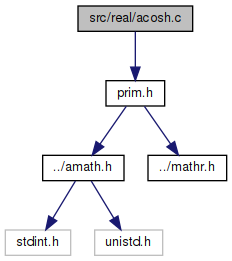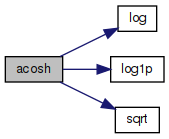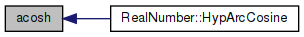amath  1.8.5 Simple command line calculator
acosh.c File Reference

Inverse hyperbolic cosine function. More...

`#include "prim.h"`
Include dependency graph for acosh.c:Go to the source code of this file.

## Functions

double acosh (double x)
Inverse hyperbolic cosine function. More...

## Variables

static const double one = 1.0

static const double ln2 = 6.93147180559945286227e-01

## Detailed Description

Inverse hyperbolic cosine function.

Definition in file acosh.c.

## ◆ acosh()

 double acosh ( double x )

Inverse hyperbolic cosine function.

```Based on
acosh(x) = log [ x + sqrt(x*x-1) ]```
```we have
acosh(x) = log(x)+ln2, if x is large; else
acosh(x) = log(2x-1/(sqrt(x*x-1)+x)) if x>2; else
acosh(x) = log1p(t+sqrt(2.0*t+t*t)); where t=x-1```
```Special cases
acosh(x) is NaN if x<1
acosh(NaN) is NaN
```

Definition at line 69 of file acosh.c.

References ln2, log(), log1p(), one, and sqrt().

Referenced by RealNumber::HypArcCosine().

70 {
71  double t;
72  int32_t hx, lx;
73
74  GET_HIGH_WORD(hx, x);
75  GET_LOW_WORD(lx, x);
76
77  // x < 1
78  if (hx < 0x3FF00000)
79  {
80  return NAN;
81  }
82
83  // x > 2**28
84  if (hx >= 0x41B00000)
85  {
86  // x is inf or NaN
87  if (hx >= 0x7FF00000)
88  {
89  return NAN;
90  }
91
92  // acosh(huge) = log(2x)
93  return log(x) + ln2;
94  }
95
96  // acosh(1) = 0
97  if (((hx - 0x3FF00000) | lx) == 0)
98  {
99  return 0.0;
100  }
101
102  // 2**28 > x > 2
103  if (hx > 0x40000000)
104  {
105  t = x * x;
106  return log(2.0 * x - one / (x + sqrt(t - one)));
107  }
108
109  // 1 < x < 2
110  t = x - one;
111  return log1p(t + sqrt(2.0 * t + t * t));
112 }
static const double one
Definition: acosh.c:49
#define GET_HIGH_WORD(i, d)
Get the more significant 32 bit int from a double.
Definition: prim.h:167
#define GET_LOW_WORD(i, d)
Get the less significant 32 bit int from a double.
Definition: prim.h:177
#define NAN
Definition: mathr.h:53
double log1p(double x)
Definition: log1p.c:122
static const double ln2
Definition: acosh.c:50
double sqrt(double x)
Square root function.
Definition: sqrt.c:119
double log(double x)
Natural logarithm function (base e)
Definition: log.c:109
Here is the call graph for this function:Here is the caller graph for this function:## ◆ ln2

 const double ln2 = 6.93147180559945286227e-01
static

Definition at line 50 of file acosh.c.

Referenced by acosh().

## ◆ one

 const double one = 1.0
static

Definition at line 49 of file acosh.c.

Referenced by acosh().